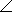# Electrical Engineering - RL Circuits

### Exercise :: RL Circuits - General Questions

11.

A 140resistor is in parallel with an inductor having 60inductive reactance. Both components are across a 12 V ac source. The magnitude of the total impedance is

 A. 5.51B. 55.15C. 90D. 200Explanation:

No answer description available for this question. Let us discuss.

12.

When the frequency of the voltage applied to a series RL circuit is decreased, the impedance

 A. decreases B. increases C. does not change D. cannot be determined without values

Explanation:

No answer description available for this question. Let us discuss.

13.

In a parallel RL circuit, there are 3 A rms in the resistive branch and 3 A rms in the inductive branch. The total rms current is

 A. 6 A B. 424 mA C. 4.24 A D. 42.4 A

Explanation:

No answer description available for this question. Let us discuss.

14.

A 47resistor is in series with an inductive reactance of 120across an ac source. The impedance, expressed in polar form, is

 A. 4768.6°B. 12068.6°C. 12931.4°D. 12968.6°Explanation:

No answer description available for this question. Let us discuss.

15.

When the resistor voltage in a series RL circuit becomes less than the inductor voltage, the phase angle

 A. increases B. decreases C. is not affected D. cannot be determined